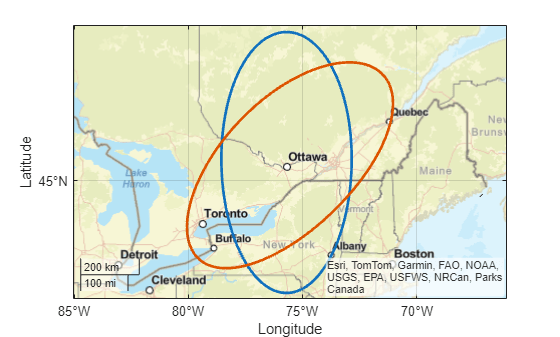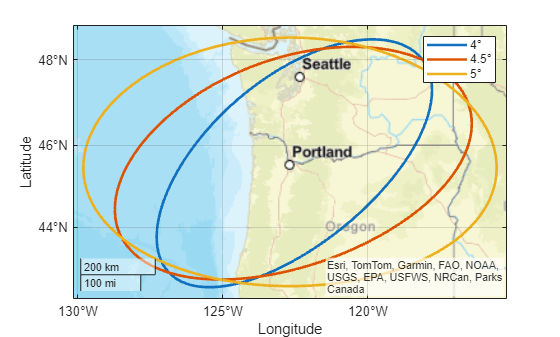# ellipse1

Geographic ellipse from center, semimajor axis, eccentricity, and azimuth

## Syntax

``[lat,lon] = ellipse1(lat0,lon0,ellipse)``
``[lat,lon] = ellipse1(lat0,lon0,ellipse,offset)``
``[lat,lon] = ellipse1(lat0,lon0,ellipse,offset,az)``
``[lat,lon] = ellipse1(lat0,lon0,ellipse,offset,az,ellipsoid)``
``[lat,lon] = ellipse1(___,units)``
``[lat,lon] = ellipse1(lat0,lon0,ellipse,offset,az,ellipsoid,units,npts)``
``[lat,lon] = ellipse1(method,___)``
``mat = ellipse1(___)``

## Description

example

````[lat,lon] = ellipse1(lat0,lon0,ellipse)` finds the latitude and longitude coordinates of an ellipse. Specify the geographic coordinates of the center of the ellipse using `lat0` and `lon0`. Specify the semimajor axis and the eccentricity of the ellipse using `ellipse`. This syntax assumes that the semimajor axis is a spherical distance in degrees. This syntax orients the ellipse so that the semimajor axis goes from north to south.```

example

````[lat,lon] = ellipse1(lat0,lon0,ellipse,offset)` rotates the ellipse using the azimuth offset `offset`.```

example

````[lat,lon] = ellipse1(lat0,lon0,ellipse,offset,az)` finds coordinates for the section of the ellipse specified by `az`.```

example

````[lat,lon] = ellipse1(lat0,lon0,ellipse,offset,az,ellipsoid)` specifies the reference ellipsoid `ellipsoid` to use for the coordinates. This syntax assumes that the semimajor axis of `ellipse` is a linear measurement in the same units as the semimajor axis of `ellipsoid`.```
````[lat,lon] = ellipse1(___,units)` specifies the angle units `units` of the inputs and outputs. You can use this syntax with any of the previous syntaxes, except `ellipse1(lat0,lon0,ellipse)`.```
````[lat,lon] = ellipse1(lat0,lon0,ellipse,offset,az,ellipsoid,units,npts)` specifies the number of points `npts` to include in the ellipse.```
````[lat,lon] = ellipse1(method,___)`, where `method` is `"rh"`, specifies that distances from the center of the small circle are rhumb line distances. The default for `method` is `"gc"`, which specifies that distances from the center of the small circle are great circle distances (for a sphere) or geodesic distances (for an ellipsoid).```
````mat = ellipse1(___)` returns the latitude and longitude coordinates of the ellipse in the matrix `mat`.```

## Examples

collapse all

Find the latitude and longitude coordinates of an ellipse centered on Ottawa with a semimajor axis of 4º and a semiminor axis of 2º. Find the eccentricity of the ellipse by using the `axes2ecc` function.

```lat0 = 45.4215; lon0 = -75.6972; semimajor = 4; ecc = axes2ecc(semimajor,2); [lat1,lon1] = ellipse1(lat0,lon0,[semimajor ecc]);```

Find the latitude coordinates of the same ellipse, this time with the semimajor axis rotated 45º from north.

`[lat2,lon2] = ellipse1(lat0,lon0,[semimajor ecc],45);`

Plot both ellipses on a map using thick lines.

```geoplot(lat1,lon1,"LineWidth",2) hold on geoplot(lat2,lon2,"LineWidth",2) geobasemap streets```Find the latitude and longitude coordinates of a full ellipse centered on Tokyo with a semimajor axis of 5º and a semiminor axis of 2º. Find the eccentricity of the ellipse by using the `axes2ecc` function.

```lat0 = 35.6762; lon0 = 139.6503; semimajor = 5; ecc = axes2ecc(semimajor,2); [lat1,lon1] = ellipse1(lat0,lon0,[semimajor ecc]);```

Find the coordinates of a partial ellipse, in this case the section of the ellipse between 90º from north and 270º from north. Avoid rotating the ellipse by specifying the fourth argument as `[]`.

```az = [90 270]; [lat2,lon2] = ellipse1(lat0,lon0,[semimajor ecc],[],az);```

Display both the full ellipse and the partial ellipse on a map. Use a thick line for the partial ellipse.

```geoplot(lat1,lon1,"Color",[0.8500 0.3250 0.0980]) hold on geoplot(lat2,lon2,"Color",[0.4940 0.1840 0.5560],"LineWidth",3) geobasemap streets```Create a World Geodetic System of 1984 (WGS84) reference ellipsoid with a length unit of kilometers.

`wgs84 = wgs84Ellipsoid("km");`

Find the latitude and longitude coordinates of an ellipse centered on Boston with a semimajor axis of 100 kilometers and a semiminor axis of 50 kilometers. Avoid rotating the ellipse and find the coordinates of the full ellipse by specifying the fourth and fifth arguments as `[]`. Find the eccentricity of the ellipse by using the `axes2ecc` function.

```lat0 = 42.3601; lon0 = -71.0589; semimajor = 100; ecc = axes2ecc(100,50); [lat,lon] = ellipse1(lat0,lon0,[semimajor ecc],[],[],wgs84);```

Plot the ellipse on a map using a thick black line.

```geoplot(lat,lon,"k","LineWidth",2) geobasemap streets```You can use the `ellipse1` function to find the coordinates of multiple ellipses with the same center or with different centers.

Multiple Ellipses with Same Center

Find the coordinates of three ellipses centered on Portland, Oregon.

• The first ellipse has a semimajor axis of 4º and a semiminor axis of 2º. Rotate the semimajor axis 50º from north.

• The second ellipse has a semimajor axis of 4.5º and a semiminor axis of 2.5º. Rotate the semimajor axis 70º from north.

• The third ellipse has a semimajor axis of 5º and a semiminor axis of 3º. Rotate the semimajor axis 90º from north.

```lat0 = 45.5152; lon0 = -122.6784; semimajor = [4 4.5 5]; ecc = axes2ecc(semimajor,[2 2.5 3]); [lat,lon] = ellipse1(lat0,lon0,[semimajor' ecc'],[50 70 90]');```

Display the ellipses on a map using thick lines. Differentiate the ellipses by including the semimajor axes in a legend.

```geobasemap streets hold on for i=1:size(lat,2) geoplot(lat(:,i),lon(:,i),"LineWidth",2,"DisplayName",string(semimajor(i))+char(176)) end legend```Multiple Ellipses with Different Centers

Find the coordinates of three ellipses with different centers.

• Center the first ellipse on Boston. Specify the semimajor axis as 4º and the semiminor axis as 2º. Rotate the semimajor axis 50º from north.

• Center the second ellipse on New York City. Specify the semimajor axis as 4.5º and the semiminor axis as 2.5º. Rotate the semimajor axis 120º from north.

• Center the third ellipse on Chicago. Specify the semimajor axis as 5º and the semiminor axis as 3º. Rotate the semimajor axis 90º from north.

```lat0 = [42.3601 40.7128 41.8781]'; lon0 = [-71.0589 -74.0060 -87.6298]'; cities = ["Boston" "New York City" "Chicago"]; semimajor = [4 4.5 5]; ecc = axes2ecc(semimajor,[2 2.5 3]); [lat,lon] = ellipse1(lat0,lon0,[semimajor' ecc'],[50 120 90]');```

Display the ellipses on a map using thick lines. Differentiate the ellipses by including the city names in a legend.

```figure geobasemap streets hold on for i=1:size(lat,2) geoplot(lat(:,i),lon(:,i),"LineWidth",2,"DisplayName",cities(i)) end legend```## Input Arguments

collapse all

Latitude of the center of the ellipse, specified as a scalar or a column vector.

• To find the coordinates of multiple ellipses with the same center, specify `lat0` and `lon0` as scalars and specify `ellipse` as a two-column matrix. The number of rows in the matrix is the number of ellipses.

• To find the coordinates of multiple ellipses with different centers, specify `lat0` and `lon0` as column vectors and specify `ellipse` as a two-column matrix. The number of elements in `lat0` and `lon0` must match the number of rows in `ellipse`.

The sizes of `lat0` and `lon0` must match.

Data Types: `double`

Longitude of the center of the ellipse, specified as a scalar or a column vector.

• To find the coordinates of multiple ellipses with the same center, specify `lat0` and `lon0` as scalars and specify `ellipse` as a two-column matrix. The number of rows in the matrix is the number of ellipses.

• To find the coordinates of multiple ellipses with different centers, specify `lat0` and `lon0` as column vectors and specify `ellipse` as a two-column matrix. The number of elements in `lat0` and `lon0` must match the number of rows in `ellipse`.

The sizes of `lat0` and `lon0` must match.

Data Types: `single` | `double`

Ellipse, specified as a two-column matrix. Each row of the matrix defines an ellipse and has the form `[semimajor_axis eccentricity]`, where `semimajor_axis` is the length of the semimajor axis and `eccentricity` is the eccentricity.

When `lat0` and `lon0` are column vectors, the number of rows in `ellipse` must match the number of elements in `lat0` and `lon0`.

Data Types: `single` | `double`

Azimuth offset of the semimajor axis, specified as a scalar or a column vector. Azimuths are measured clockwise from north. The default value of `[]` indicates that the semimajor axis goes from north to south.

Unless `offset` is `[]`, the size of `offset` must match the number of ellipses.

Data Types: `single` | `double`

Azimuth indicating the section of the ellipse to find, specified as one of these options:

• A column vector — Find coordinates of the ellipse from north to the specified azimuth. Each element of `az` corresponds to a row of `ellipse`. The size of `az` must match the number of ellipses.

• A two-column matrix — Find coordinates of the ellipse between the azimuth in the first column and the azimuth in the second column. Each row of `az` corresponds to a row of `ellipse`. The number of rows in `az` must match the number of ellipses.

Azimuths are measured clockwise from north.

The default value of `[]` specifies a full ellipse.

Data Types: `single` | `double`

Reference ellipsoid, specified as a `referenceSphere` object, a `referenceEllipsoid` object, an `oblateSpheroid` object, or a two-element numeric vector of the form `[semimajor_axis eccentricity]`, where `semimajor_axis` is the length of the semimajor axis and `eccentricity` is the eccentricity. The values `semimajor_axis` and `eccentricity` must be of data type `double`.

The default value of `[1 0]` represents the unit sphere.

Angle unit, specified as one of these options:

• `"degrees"` — Degrees

• `"radians"` — Radians

If you do not specify a reference ellipsoid, this argument determines the angle units for the coordinates, `offset`, `az`, and the semimajor axis of `ellipse`. If you specify a reference ellipsoid, this argument determines the angle units for only the coordinates, `offset`, and `az`.

Data Types: `char` | `string`

Number of points to include in the ellipse, specified as a scalar. If you specify more than one ellipse, then the function finds `npts` points per ellipse.

Data Types: `single` | `double`

Type of distance from the center of the ellipse, specified as one of these options:

• `"gc"` — Use the great circle distance.

• `"rh"` — Use the rhumb line distance.

Data Types: `char` | `string`

## Output Arguments

collapse all

Latitude coordinates of the ellipse, returned as a column vector with `npts` elements or a matrix of size `npts`-by-m, where m is the number of ellipses.

Longitude coordinates of the ellipse, returned as a column vector with `npts` elements or a matrix of size `npts`-by-m, where m is the number of ellipses.

Latitude and longitude coordinates of the ellipse, returned as a matrix equivalent to `[lat lon]`.

## Version History

Introduced before R2006a